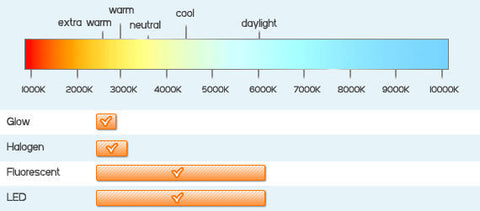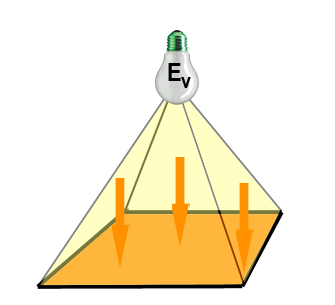# Lighting Definitions

Luminous Flux

Luminous flux is the quantity of the energy of the light emitted per second in all directions. The unit of luminous flux is lumen (lm). One lumen is the luminous flux of the uniform point light source that has luminous intensity of 1 candela and is contained in one unit of spatial angle (or 1 steradian). Steradian is the spatial angle that limits the surface area of the sphere equal to the square of the radius. This concept is shown in the figure for 1 m radius of the sphere. Since the area of sphere is 4pr² then the luminous flux of the point light source is 4p lumensLuminous Intensity

Luminous intensity is the ability to emit light into a given direction, or it is the luminous flux that is radiated by the light source in a given direction within the unit of the spatial angel. If the point light source emits Ø lumens into a small spatial angel ß, the luminous intensity is I=Ø/ß.
The unit of luminous intensity is candela. There is a standard that details the candela definition. This includes the standard light source and the physical conditions of the measurement.

Illuminance (Illumination)

This definition determines the amount of light that covers a surface. If Ø is the luminous flux and S is the area of the given surface then the illuminance E is determined by E=Ø/S.

The unit of illumination in SI system is lx, and in foot-pound system it is foot-candle. One lx is the illuminance of 1 m² surface area uniformly lighted by 1 lm of luminous flux. The following drawing explains this definition. One foot-candle is 10.76 lux.

Light colour

Light colours, like cool white and warm white, tell us what kind of light a certain lamp will spread. These light colours are determined by their colour temperature, which is indicated in Kelvin. If a lamp has a low Kelvin value, its light will be more red (warm), higher values will give off a more blueish light (cool).

Candle light for example is somewhere around 2000K, while a standard halogen bulb will be in between 2800K and 3000K. Below you can find the different light colours you can become by using the most common light sources.Luminance

Luminance L is the luminous intensity emitted by the surface area of 1 cm² (or 1 m²) of the light source. Mathematiclly it is L=I/S where I is the luminous intensity and S is the area of the source surface perpendicular to the given direction.
The unit of luminance is cd/m² or cd/cm² (in some applications lm/cm² or Lambert can be used). The following figure shows the concept.